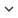ENGArticle
Article 459
Author/s
• Matabuena M., Rodríguez-López R.
DOI
Source
• Bulletin of Mathematical Biology, 2019 - Q1

# An Improved Version of the Classical Banister Model to Predict Changes in Physical Condition

© 2019, Society for Mathematical Biology. In this paper, we formulate and provide the solutions to two new models to predict changes in physical condition by using the information of the training load of an individual. The first model is based on a functional differential equation, and the second one on an integral differential equation. Both models are an extension to the classical Banister model and allow to overcome its main drawback: the variations in physical condition are influenced by the training loads of the previous days and not only of the same day. Finally, it is illustrated how the first model works with a real example of the training process of a cyclist.
Keywords: Difference equations, Functional differential equations, Mathematical models